Recently there has been a great deal of interest in Lead-free solders. A number of metallurgical factors are important in evaluating the utility of the alternatives to the standard Lead-Tin solder on various substrates. Another aspect that is important is how well the molten solder wets a given substrate. If the solder doesn't wet the substrate or wire at all, then no joint can be formed. Too much wetting can lead to shorting, for example.

A standard test for evaluating the wetting characteristics of solder is an "area of spread test", where a drop of molten solder is allowed to spread on a flat substrate. The rate and extent of the spreading are observed. Such experiments have been carried out in the Metallurgy Division at NIST. We have attempted to mathematically model some aspects of these experiments.

One effect that we believe may be important is the Marangoni effect, where concenctration and temperature gradients induce shear stresses on the free surface of the drop. Because the molten solder reacts with the metal substrate, concentration gradients may exsit in the drop that create shear stresses on the free surface of the drop that in turn modify the fluid velocity in the drop. For typical solder materials, the parameters are such that the fluid motion strongly affects the distribution (concentration) of the alloying elements.

## Finite Element Modeling

Some graphical results of finite element models of the flows in drops are show below; they were obtained using a commercial finite element package. The Schmidt number a material parameter which is the ratio of the momentum and solutal diffusivities; this number is typically large for solder materials (for Lead-Tin, this ratio is 81). The Prandtl number (ratio of momentum and thermal diffusivities) is a second material parameter which is typically small for liquid metals. The first figure shows the temperature field of a drop with a Prandtl number of 0.08 which is heated from below; the essentially linear temperature field is unaffected by the streamlines shown in the second figure. The streamlines illustrate a case where a single vortex is being driven by Marangoni effects and there is no (further) spreading.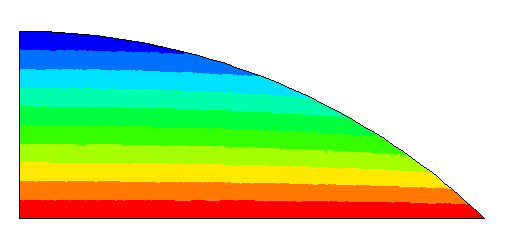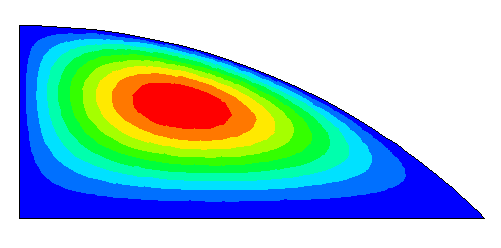Below are two different results for Schmidt numbers of 1 and 20. The reaction with the substrate lowers the concentration at the base (blue); the concentration field is strongly affected by the flow.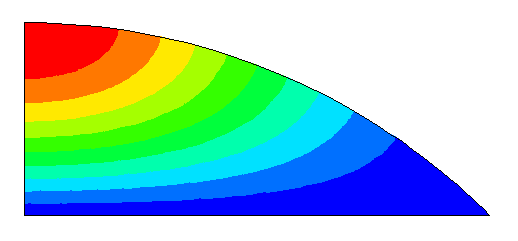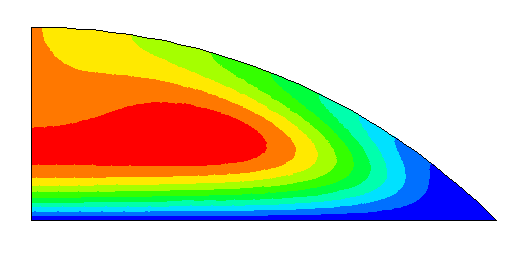The results demonstrate the large effect of flow on solute transport when the Schmidt number is large.

## Lubrication Theory

The previous results shown are obtained using a method that is not well suited to extreme changes in geometry. Spreading of solder drops may involve extreme changes of geometry; some of the final angles when the drops stop spreading are just 3 degrees. An approach that is better suited to very thin drops is lubrication theory, which is a multiple-scale asymptotic approach. Using lubrication theory, we have derived nonlinear evolution equations for the free surface of the drop and the concentration field within the drop. The evolution equations are solve using a variation of the method of lines where we collocate on Chebyshev points and solve the resulting system of ordinary differential and algebraic equations using the package DASSL. Some results are shown below.

In each figure, the top drop shape has contours of concentration, while the bottom one has streamlines. The first figure is the initial concentration distribution and streamfunction. In all of these results the drop is heated from below, resulting in an essentially linear temperature distribution (not shown) similar to the one shown above. In the first figure, the contours indicate jumps of 0.1 in concentration and 0.01 in the streamfunction.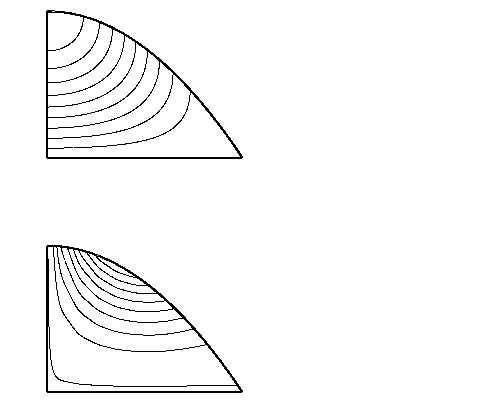In the second figure, the contours indicate jumps of 0.1 in concentration (upper drop) and 0.00002 in the streamfunction (lower drop). The dimensionless time is 22.0.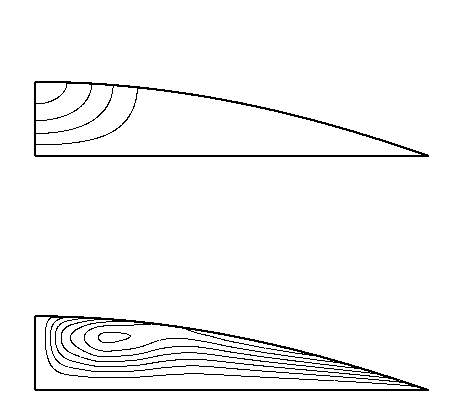In the third figure, the contours indicate jumps 0.000005 in the streamfunction (lower drop). The concentration is essentially constant. The dimensionless time is 7071., and the spreading is about 86% complete.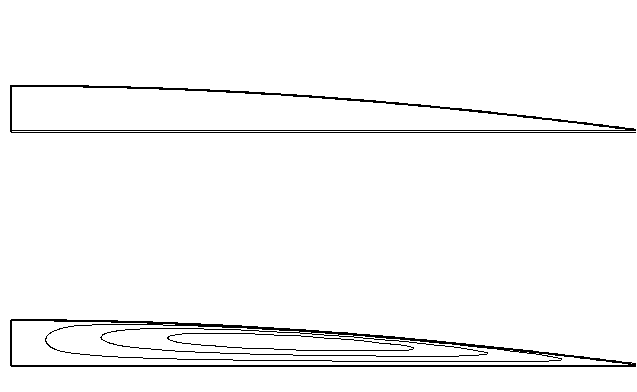While these figures show that lubrication theory is well suited to the large geometry changes which may occur in spreading, the results presented are only valid for a fluid with a Schmidt number of about 1. The lubrication theory results must be extended to reach the parameter regime of real solder materials.